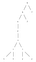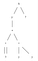# Part 1: The Nix expression language

`\$ nix-instantiate --eval --expr '"Hello world"'"Hello world"`
`"Hello world"`
`"Hello world"`
`Expression ::= StringString     ::= '"' StringChar* '"'StringChar ::= Space | Alphanumeric`
`\$ nix-instantiate --eval --expr "'Hello world'"error: syntax error, unexpected \$undefined, at (string):1:1`

## Special characters

`> "He said "Hello world""error: undefined variable `Hello' at (string):1:11`
`> "He said \"Hello world\"""He said \"Hello world\""`
`> "Write \\\" to write a literal double-quote""Write \\\" to write a literal double-quote"`
`StringChar ::= '\\' | '\"' | Space | Alphanumeric`
`Sequence    Encodes            Character          Unicode code point----------  -----------------  ------------------\n          line feed          10\t          tab                11\r          carriage return    13\\          backslash          92`

## Primitive types and operators

`\$ nix-instantiate --eval --expr '42'    # integers42\$ nix-instantiate --eval --expr 'true'  # booleanstrue`
`\$ nix-instantiate --eval ––expr '"Hello " + "world"'"Hello world"\$ nix-instantiate --eval ––expr '2 + 3'5`
`\$ nix-instantiate --eval ––expr '(400 + 2) * (-5) + (5 * 30)'-1860\$ nix-instantiate --eval --expr '(4 * 4 * 4) < (5 * 5 * 5)'true`
`\$ nix-instantiate --eval --expr '2/3'/Users/jhf/dev/nix/2/3`
`\$ nix-instantiate --eval --expr '2 / 3'0`

## REPL

`> (4 * 4 * 4) < (5 * 5 * 5)true`
`\$ nix-env -i nix-repl\$ nix-replnix-repl> 4*4*4 < 5*5*5true`

## Errors

`> "Hello" + 6error: cannot coerce an integer to a string, at (string):1:1`
`> abort "Just not feeling it today"error: evaluation aborted with the following error message: `Just not feeling it today'`

## Typing discipline

`> builtins.typeOf "foo""string"> builtins.typeOf (2 + 2)"int"> builtins.typeOf ("foo" + 2)error: cannot coerce an integer to a string, at (string):1:18`
`> builtins.isInt (2 + 2)true> builtins.isBool "true"false> builtins.isBool falsetrue`
`# 6 : int# builtins.isInt : any -> bool# builtins.isInt 6 : bool# builtins.typeOf : any -> string`

## Function application

`> builtins.isInt 4true> builtins.isInt(4)true`
`> builtins.div 10 52`
`> (builtins.div 10) 52`
`> builtins.typeOf   (builtins.div)"lambda"> builtins.typeOf  ((builtins.div) 10)"lambda"> builtins.typeOf (((builtins.div) 10) 5)"int"`
`# builtins.div : int -> int -> int`
`# builtins.div : int -> (int -> int)`

## Function definition

`> x: x*x     # int -> int<LAMBDA>`
`> builtins.typeOf (x: x*x)"lambda"`
`> (x: x*x) 39`
`> (x: y: x*x + y*y)   # int -> int -> int<LAMBDA>> (x: y: x*x + y*y) 3 758`

## Parse trees and evaluation order

`((x: (y: (x*x + y*y))) 3) 7`Initial abstract syntax tree represented by our input program.After one step. We replaced all references to x with references to 3.
`((x: (y: (x*x + y*y))) 3) 7    # initial expression(y: (3*3 + y*y)) 7             # substitute x=33*3 + 7*7                      # substitute y=79 + 7*7                        # multiplication9 + 49                         # multiplication58                             # addition`

## Let expressions

`> (square: (x: y: square x + square y) 3 7)  (x: x*x)58`
`> let square=(x: x*x); in (x: y: square x + square y) 3 758`

## Detour: evaluating files

`let # square : int -> int square = x: x*x; # sumOfSquares : int -> int -> int sumOfSquares = x: y: square x + square y;in sumOfSquares 3 7`
`\$ nix-instantiate --eval squares.nix58`
`let square = x: x*x; sumOfSquares = x: y: square x + square y;in sumOfSquares 3 7==> 58`

## Conditionals

`let  # abs : int -> int abs = x:    if x < 0 then (-x)            else x;in abs (-3)==> 3`
`(x: if x < 0 then (-x) else x) (-3)  # initial expressionif (-3) < 0 then (-(-3)) else (-3)   # function applicationif true then (-(-3)) else (-3)       # do less-than operator(-(-3))                              # do conditional3                                    # do negation`

## Boolean operators and laziness

`Operator expression         Equivalent to! A                         if A then false else trueA || B                      if A then true else BA && B                      if A then B else falseA -> B                      if A then B else true`
`> false && (abort "hmm")false> true && (abort "hmm")error: evaluation aborted with the following error message: `hmm'`

## Recursion

`let # factorial : int —> int factorial = n: if n == 0 then 1                          else n * factorial (n — 1);in factorial 5==> 120`

## Loops?

`let # fib' : int -> int -> int -> int fib' = i: n: m:  if i == 0 then n                            else fib' (i — 1) m (n + m); # fib : int -> int  fib = n: fib' n 1 1;in fib 30==> 1346269`

## Composite data-types and laziness

`> { "name" = "james"; age = 26; }{ age = 26; name = "james"; }`
`> { age = 2014 — 1988; }{ age = <CODE>; }`
`\$ nix-instantiate --eval --expr --strict '{ age = 2014-1987; }'{ age = 27; }`
`nix-repl> :p { age = 2014-1987; }{ age = 27; }`
`> { age = 2014–1987; }.age27`
`{ age = 2014–1987; }.age   # 1. initial expression2014–1987                  # 2. do the dot operator26                         # 3. do the subtraction operator`
`{ age: 2014–1987 }.age     # 1. initial expression{ age: 26 }.age            # 2. do the subtraction operator26                         # 3. do the dot operator`

## Lists

`> [1 (1+1) "three"]        # list any[ 1 <CODE> "three" ]`
`> builtins.elemAt [1 (1+1) "three"] 12`
`> builtins.attrNames { age = 26; name = "james"; }[ "age" "name" ]> builtins.tail [1 2 3 4 5][ 2 3 4 5 ]> builtins.head [1 2 3 4 5]1> [1 2 3] ++ [4 5 6][ 1 2 3 4 5 6 ]`

## Infinite data structures

`let  # fibsFrom : int -> int -> list int  fibsFrom = n: m: [n] ++ fibsFrom m (n+m);    # fibs : list int  fibs = fibsFrom 1 1;in  builtins.elemAt fibs 30==> segmentation fault`
`# type stream a = U nil { head=a; tail=stream a; } let  # streamElemAt = s: i:    if i == 0 then s.head              else streamElemAt s.tail (i - 1);  fibsFrom = n: m: {    head = n;    tail = fibsFrom m (n + m);  };  fibs = fibsFrom 1 1;in  streamElemAt fibs 30==> 1346269`

## Lazy loopiness and recursive sets

`let  james = { surname = dad.surname; age = 26;             };  dad   = { surname = "fisher";    age = james.age + 28; };in  { james = james; dad = dad; }==(strict evaluation)==> {  dad =   { age = 54; surname = "fisher"; };  james = { age = 26; surname = "fisher"; };}`
`rec { james = { surname = dad.surname; age = 26;             }; dad   = { surname = "fisher";    age = james.age + 28; };}`

## Modules, public and private

`let  cons    = head: tail: { head = head; tail = tail; };  iterate = step: head: cons head (iterate step (step head));  map     = f: {head, tail}: cons (f head) (map f tail);  elemAt  = l: i: if i == 0 then l.head                            else elemAt l.tail (i - 1);in {  iterate = iterate;  map = map;  elemAt = elemAt;}`

## Imports and paths

`> /etc/passwd/etc/passwd> builtins.typeOf /etc/passwd"path"`
`> ./foo.nix/Users/jhf/dev/nix/foo.nix`
`> foo.nixerror: undefined variable `foo' at (string):1:1`
`> ./streams.nix/Users/jhf/dev/nix/streams.nix`
`let  fibsFrom = n: m: {    head = n;    tail = fibsFrom m (n + m);  };    fibs = fibsFrom 1 1;in  (import ./streams.nix).elemAt fibs 30==> 1346269`

## Circular imports

`{ surname = (import ./dad.nix).surname; age = 26; }`
`{ surname = "fisher"; age = (import ./james.nix).age + 28; }`
`> { james = import ./james.nix; dad = import ./dad.nix; }{ dad = { age = 54; surname = "fisher"; }; james = { age = 26; surname = "fisher"; }; }`

## Dynamic scope

`with (import ./streams.nix);let fibsFrom = n: m: {   head = n;   tail = fibsFrom m (n + m); };  fibs = fibsFrom 1 1;in elemAt fibs 30==> 1346269`

## Multi-parameter functions using sets

`> (x: y: x*x + y*y) 3 758> (args: args.x*args.x + args.y*args.y) {x=3; y=7;}58`
`> ({x, y, ...}: x*x + y*y) {x=3; y=7;}58`
`> ({x, y}: x*x + y*y) {x=3;y=7;z=9;}error: anonymous function at (string):1:2 called with unexpected argument `z’, at (string):1:1`

## Default arguments

`(args: let   # builtins.hasAttr : string -> set -> bool   x = if builtins.hasAttr "x" args then args.x else 0;   y = if builtins.hasAttr "y" args then args.y else 0; in (x * x) + (y * y)) {x=3;}==> 9`
`(args':  let args = { x=0; y= 0; } // args';      # // is not a comment!  in (args.x * args.x) + (args.y * args.y)) {x=3;}==> 9`
`> ({x ? 0, y ? 0}: (x * x) + (y * y)) {x=3;}9`

## Exceptions

`> throw "I'm an exception"error: I'm an exception`
`> builtins.tryEval (throw "I'm an exception"){ success = false; value = false; }`
`> builtins.tryEval (2 + 2){ success = true; value = 4; }`
`> builtins.tryEval (abort "I'm an error")error: evaluation aborted with the following error message: `I'm an error'`

## Assertions

`> assert (2 < 1); "icecream"error: assertion failed at (string):1:1> assert (1 < 2); "icecream""icecream"`
`let max = x: y:   assert builtins.isInt x;   assert builtins.isInt y;   if x < y then y            else x;in  max 5 "six"==> error: assertion failed`
`let max = x: y:   let     attempt = builtins.tryEval (       assert builtins.isInt x;       assert builtins.isInt y;       if x < y then y                else x     );   in     if attempt.success then attempt.value                        else throw "max : int -> int -> int";in  max 5 "six"==> error: max : int -> int -> int`

## Debugging

`builtins.trace : forall a b. a -> b -> b`
`> builtins.trace 1 2trace: 12`
`> { foo = builtins.trace 1 2; }{ foo = <CODE>; }`
`> builtins.trace { foo = 2 + 2; } "foo"trace: { foo = <CODE>; }"foo"`

## Next time …

I don’t blog here any more; see https://jameshfisher.com. Also see Vidrio for Mac, the holographic presentation app from the future: https://vidr.io

## More from James Fisher

I don’t blog here any more; see https://jameshfisher.com. Also see Vidrio for Mac, the holographic presentation app from the future: https://vidr.io